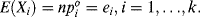# 13.4 Testing Goodness Of Fit

Having discussed the particulars of the chi-square distribution, let us return to the use of the test statistic (Eq. 13.4). Why is this test statistic actually structured to reveal goodness of fit? It should be intuitively clear that the notion of goodness of fit should be assessed on the basis of the degree of disparity between the sample or empirical distribution and the expected or theoretical distribution given that the latter is specified by the null hypothesis. Under H0, the expected or theoretical frequencies for the k categories or cells are simplyThat is, ei is the product between the sample size and the hypothesized relative frequency or theoretical probability. So if H0 is true, ei = nshould be the expected number of occurrences for cell i under n repeated trials of our k-fold alternative experiment. The expected cell frequencies are given in column 4 of Table 13.1. Under this discussion, we may modify Equation (13.4) to read

(13.5)Here U0 serves as an index of goodness of fit given that H0 specifies the theoretical distribution that is fitted ...

Get Statistical Inference: A Short Course now with the O’Reilly learning platform.

O’Reilly members experience live online training, plus books, videos, and digital content from nearly 200 publishers.